Courses

Electrostatic MCQ (With Solution)Level - 1 : Test 2

30 Questions MCQ Test | Electrostatic MCQ (With Solution)Level - 1 : Test 2

Description
Attempt Electrostatic MCQ (With Solution)Level - 1 : Test 2 | 30 questions in 60 minutes | Mock test for Class 12 preparation | Free important questions MCQ to study for Class 12 Exam | Download free PDF with solutions
QUESTION: 1

Two small sphere balls each carrying charge q = 10µC are suspended by two insulated threads of equal length 1m each, from a point fixed in the ceiling. It is found that in equilibrium, threads are separated by an angle 600 between them as shown in figure, the tension in the thread is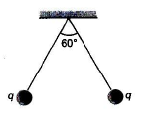Solution:

Let tension in the thread is T, then force of repulsion between the charges.
F = T cos 60°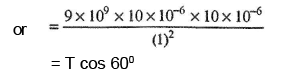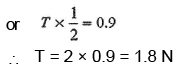QUESTION: 2

The charge on two identical metalic balls are +40µC and -10µC respectively and they are separated at 2.0m.How much and nature of force will act between them?

Solution: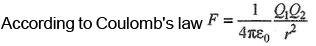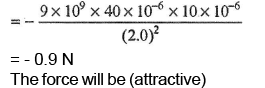QUESTION: 3

If a body is charged by rubbing it, its weight

Solution:

The body may slightly get charged either negatively or positively.

QUESTION: 4

In S.I system, the value of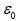is

Solution: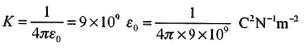QUESTION: 5

Dimensions ofare

Solution: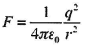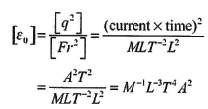QUESTION: 6

The dielectric constant K of an insulator can be :

Solution:

Dielectric constant of a material is K > 1.

QUESTION: 7

Choose the correct statement :

Solution:

Net charge of universe is constant. Positive and nagative charges may separately be created or destroyed. But net sum of charge remains constant.

QUESTION: 8

Two neutrons are placed at some distance apart from each other. They will

Solution:

There will be gravitational attraction between the neutrons.

QUESTION: 9

There are two charges +1 µC and +5 µC. The ratio of the forces (force on one due to other) acting on them will be

Solution:

Newton's third law.

QUESTION: 10

Five balls numbered 1, 2, 3, 4, 5 are suspended using separate threads. The balls (1, 2), (2, 4) and (4, 1) show electrostatic attraction, while balls (2,3) and (4, 5) show repulsion, Therefore, ball 1 must be

Solution: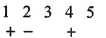If 1 is positively charged, then 2 should be negatively charged, then 4 should be positively charged. Now, 1 and 4 cannot attract. It means ball 1 should be neutral (2 and 4 cannot be neutral because they are showing repulsion with 3 and 5, respectively).

QUESTION: 11

Electric charges A and B repel each other, Electric charges B and C also repel other. If A and C are held close together, they will.

Solution:

A and B have like charges, and B and C have like charges. So, A and C also have like charges. Hence, they also repel each other.

QUESTION: 12

Two point charges repel each other with a force of 100 N One of the charges is increased by 10% and the other is reduced by 10%. The new force of repulsion at the same distance would be

Solution: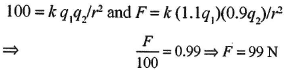QUESTION: 13

Two positive and equal charges are fixed at a certain distance. A third small charge is placed in between the two charges and it experiences zero net force due to the other two.

Solution:

The equilibrium can be stable only if on displacing the small charge slightly in any direction, the forces act on it in such a way so as to bring back the charge to its equilibrium position.

QUESTION: 14

An isolated charge q1 of mass m is suspended freely by a thread of length l. Another charge q2 is brought near it (r>> l). W hen q1 is in equilibrium, tension in thread will be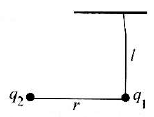Solution:

Initial tension : T1 = mg
Final tension : T2 cos θ = mg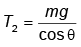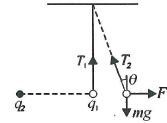Obviously, T2 > mg.
Here we have assumed θ to be small so that F is almost horizontal.

QUESTION: 15

A point charge q1, is moved along a circular path of radius r in the electric field of another point charge q2 at the centre of the parh. The work done by the electric field on the charge q1 in half revolution is

Solution:

As the force's always perpendicular, therefore work done is always zero.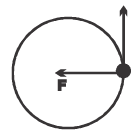QUESTION: 16

A spherical conducting ball is suspended by a grounded conducting thread. A positive point charge is moved near the ball. The ball will

Solution:

It will get attracted due to induction.

QUESTION: 17

Five point charges, each of value +q, are placed on five vertices of a regular hexagon of side L. The magnitude of the force on a point charge of value -q coulomb placed the centre of the hexagon is

Solution:

If all charges were present force on central charge will be zero.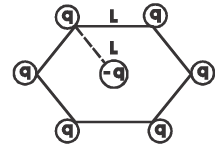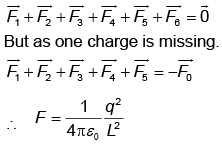QUESTION: 18

The sure test of electrification is :

Solution:

Attraction m ay take place between charged and uncharged body too. So, repulsion is the surest test of charge.

QUESTION: 19

The number of electrons in one coulomb of charge will be :

Solution: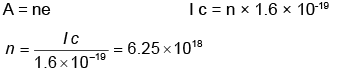QUESTION: 20

The minimum value of charge on any charged body may be :

Solution:

Minimum charge is the electronic charge.

QUESTION: 21

W hen 1014 electrons are removed from a neutral metal sphere, the charge on the sphere becomes

Solution:

Charge on the body will be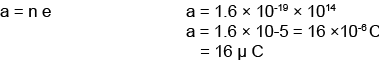QUESTION: 22

One metallic sphere A is given a positive charge whereas another metallic sphere B of exactly the same mass is given a negative charge. Then

Solution:

Positively charged body loses some mass whereas negatively charged body gains some mass.

QUESTION: 23

An electron has charge 1.6 × 10-19 coulomb when at rest. When it moves with speed 0.8c the charge on the electron would be (c being speed of light in vacuum)

Solution:

Charge is invarient in nature.

QUESTION: 24

A charged rod attracts bits of dry cork dust which after touching the rod

Solution:

On touching it shares charge and then idential charges repel.

QUESTION: 25

The electrostatic force between two point charges q1 and q2 at separation r is given by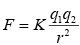The constant K

Solution: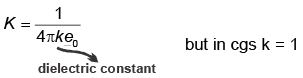So k depends on medium as well as system of units.

QUESTION: 26

A charge q1 exerts some force on a second charge q2.If third charge q3 is brought near, the force of q1 exerted on q2

Solution:

Due to principle of superposition force will not depend upon presence of another charge.

QUESTION: 27

If Fe and Fg are electric and gravitational force, respectively between two electrons situated at certain distance apart, then the ratio of Fe and Fg is of the order of

Solution: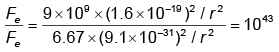QUESTION: 28

A thin stream of water from a tap is attracted by placing a rod near it. The rod is

Solution:

The charge on rod attracts the stream of water due to induction.

QUESTION: 29

The proton is 1836 times heavier than electron. The Coulomb's force of repulsion between two protons at a certain distance between them is F. Then the Coulomb force between two electrons for same distance would be :

Solution:

Coulomb's force does not depend upon mass.

QUESTION: 30

The electric field intensity at a point situated 4 meters from a point charge is 200 N/C. If the distance is reduced to 2 meters, the field intensity will be

Solution:Use Code STAYHOME200 and get INR 200 additional OFF Use Coupon Code

Track your progress, build streaks, highlight & save important lessons and more!

Similar ContentRelated tests